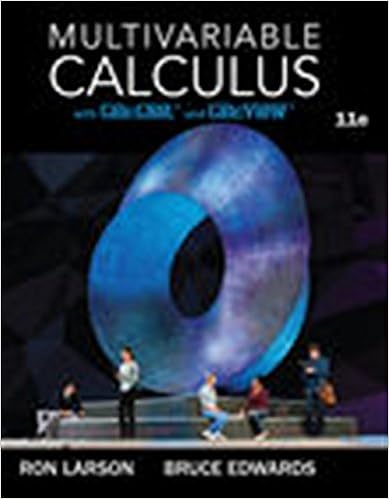# math 260 lab 5 - MATH260Week 5 Lab Name Kong Lee Category 1...

• Lab Report
• 7
• 86% (22) 19 out of 22 people found this document helpful

This preview shows page 1 - 2 out of 7 pages.

##### We have textbook solutions for you!
The document you are viewing contains questions related to this textbook.The document you are viewing contains questions related to this textbook.
Chapter 13 / Exercise 36
Multivariable Calculus
LarsonExpert Verified
MATH260—Week 5 LabName: Kong LeeCategory 1: Derivatives of Exponentials: eDirections:Look at the examples below and answer the question.f(x) = exf (x) = ef(x) = e5xf (x) = 5e5xf(x)=4ex2f '(x)=8x ex2f(x)=ex32xf '(x)=(3x22)ex32x1) Describe in your own words what to do to find the derivative for problems like those above. Write the original argument and multiply the power to get the answer for a first derivativeof the original argument. ux
2) Describe in your own words what to do to find the derivative for problems like those above
##### We have textbook solutions for you!
The document you are viewing contains questions related to this textbook.The document you are viewing contains questions related to this textbook.
Chapter 13 / Exercise 36
Multivariable Calculus
LarsonExpert Verified
•••